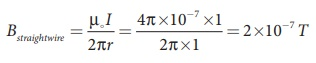Home | | Physics 12th Std | Ampere’s Circuital Law: Solved Example Problems

# Ampere’s Circuital Law: Solved Example Problems

Physics : Magnetism and Magnetic Effects of Electric Current: Ampere’s Circuital Law: Solved Example Problems with Answers, Solution and Explanation

## Magnetic field due to the current carrying wire of infinite length using Ampère’s law : Solved Example Problems

EXAMPLE 3.18

Compute the magnitude of the magnetic field of a long, straight wire carrying a current of 1A at distance of 1m from it. Compare it with Earth’s magnetic field.

Solution

Given that 1 = 1 A and radius r = 1 mBut the Earth’s magnetic field is BEarth  ≈ 10−5 T

So, Bstraightwire is one hundred times smaller than BEarth.

## Magnetic field due to a long current carrying solenoid: Solved Example Problems

EXAMPLE 3.19

Calculate the magnetic field inside a solenoid, when

(a) the length of the solenoid becomes twice and fixed number of turns

(b) both the length of the solenoid and number of turns are double

(c) the number of turns becomes twice for the fixed length of the solenoid

Compare the results.

Solution

The magnetic field of a solenoid (inside) is(a) length of the solenoid becomes twice and fixed number of turns

L→2L (length becomes twice)

N→N (number of turns are fixed)

The magnetic field is

B2L , N = µ NI/2L = 1/2 BL ,N

(b) both the length of the solenoid and number of turns are double

L→2L (length becomes twice)

N→2N (number of turns becomes twice)

The magnetic field is(c) the number of turns becomes twice but for the fixed length of the solenoid

L→L (length is fixed)

N→2N (number of turns becomes twice)

The magnetic field is

BL ,2 N = µ, 2NI/L = 2BL ,N

From the above results,

BL ,2 N > B2 L ,2 N > B2 L , N

Thus, strength of the magnetic field is increased when we pack more loops into the same length for a given current.

Tags : Physics , 12th Physics : Magnetism and Magnetic Effects of Electric Current
Study Material, Lecturing Notes, Assignment, Reference, Wiki description explanation, brief detail
12th Physics : Magnetism and Magnetic Effects of Electric Current : Ampere’s Circuital Law: Solved Example Problems | Physics